# PERCENTILE Function

Returns the k-th percentile of the values in a particular range

## What is the PERCENTILE Function?

The PERCENTILE function is categorized under Excel Statistical functions. PERCENTILE will return the k-th percentile of the values in a particular range.

In corporate finance, we can use the function to analyze, for example, the number of employees who scored above a certain percentile on a test.

In MS Excel 2010, the PERCENTILE function was replaced by the PERCENTILE.INC function. However, PERCENTILE is still available in current versions of Excel (stored in the list of compatibility functions).

### Formula

=PERCENTILE(array,k)

The PERCENTILE function uses the following arguments:

1. Array (required argument) – This is the array or range of data that defines the relative standing.
2. k (required argument) – The percentile value in the range 0…1, inclusive.

### How to use the PERCENTILE Function in Excel?

As a worksheet function, PERCENTILE can be entered as part of a formula in a cell of a worksheet. To understand the uses of the function, let’s consider an example:

#### Example

Suppose we are given the following data: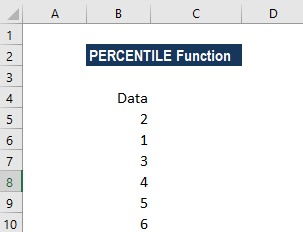We will use several formulas to get what we want to know. The first formula we use is PERCENTILE(B5:B10,0.1):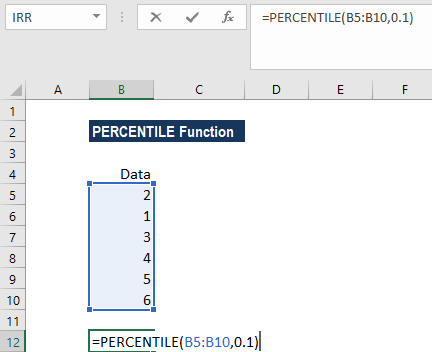We get the results below: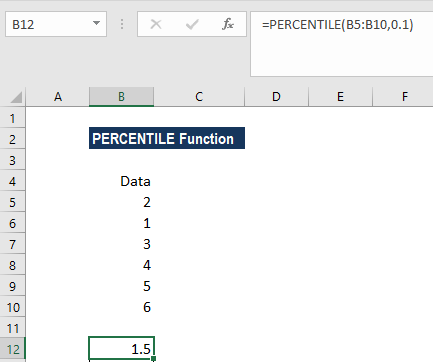The value of k can be entered as a decimal or a percentage. Here, the value 0.1 in cell B12 is the same as 10% (the 10th percentile).

If we use the formula PERCENTILE(B5:B10,50%), as shown below: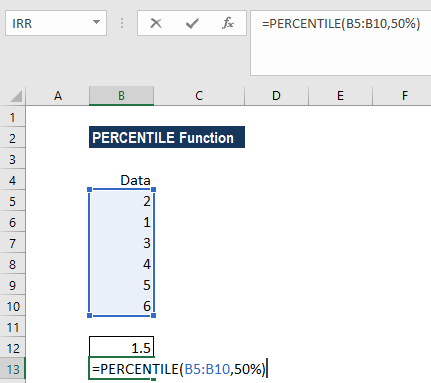Then we get the result below: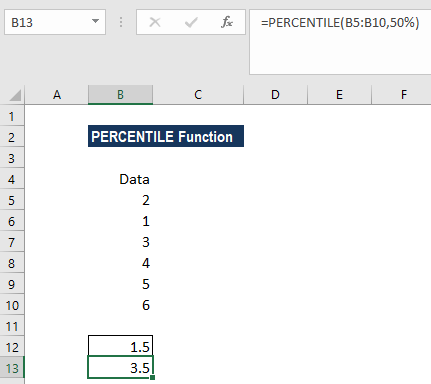Here, the 50th percentile in cell B13 falls halfway between the values of 3 and 4. Therefore, Excel interpolated to produce the result of 3.5.

### A few notes about the PERCENTILE function:

1. #NUM! error – Occurs if either:
1. The supplied value of k is less than 0 or greater than 1.
2. The supplied array is empty.
2. #VALUE! error – Occurs if the supplied value of k is non-numeric.
3. If k is not a multiple of 1/(n – 1), PERCENTILE interpolates to determine the value at the k-th percentile.

Thanks for reading CFI’s guide to this useful Excel function. By taking the time to learn and master these functions, you’ll significantly speed up your financial analysis and Excel modeling. To learn more, check out these additional CFI resources:

• Financial modeling guide